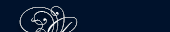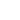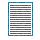НОВОСТИ   БИБЛИОТЕКА   ЭНЦИКЛОПЕДИЯ   КАРТА САЙТА   ССЫЛКИ   О САЙТЕ## PIERRE DE FERMAT

Pierre de Fermat lived in Toulouse and was a councillor at the Parliament of Toulouse. He was interested in arithmetical questions and his duties did not prevent him from his mathematical research.

Fermat was one of those who participated in the origins of infinitesimal calculus and in the creation of calculus of probabilities.

Fermat knew the works of ancient mathematicians. After his death a translation of the work of Diophantes, a Greek mathematician, was found in Fermat's papers. There was an observation in Latin in the margin of the book: “I have proved that the relation xm + ym = zm is impossible in integral numbers (x, y, z, different from 0; m greater than 2)., but the margin does not leave me room enough to inscribe the proof” and Fermat was not mistaken.

Fermat's immense work made it possible to get partial proofs of it. Three centuries have passed since then, and the scientists are still looking for the proof which Fermat had not written in the margin.

calculus of probabilities исчисление вероятностей

Diophantes Диофант

infinitesimal calculus исчисление бесконечно малых

Pierre de Fermat Пьер де Ферма

room enough достаточно места

Toulouse г. Тулуза

xm + ym = zmis read x in m (power) plus у in m (power) equals z in m.ПОИСК: